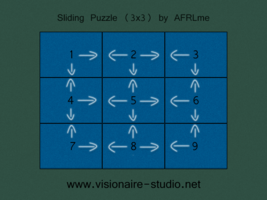# Sliding Puzzle (CMS)

(diff) ← Older revision | Latest revision (diff) | Newer revision → (diff)
Name Type By
Sliding Puzzle (3x3) Definition AFRLme

This script is used to generate & control sliding puzzle consisting of a 3x3 grid. Visionaire Studio 5.1.9.1 + is required to run this script. This script is just a basic example of a sliding puzzle, you should be able to easily modify it for any grid size. It should also be possible to generate from a randomized tile order providing you provide a table of known working tile positions. You could also add a move counter, or even limit the amount of moves that can be made if you want to make the puzzle harder.

Check out this website here, for more in depth information on sliding puzzle design.

## Instructions

1. Add the main script to the Visionaire Studio Script Editor & set the script as a definition script.
2a. First thing you should do is update the t to t tables with the correct x, y positions for where each tile should be placed (top left pixel).

``` t = { .. x = 288, y = 221
```

2b. The second thing you need to do is specify the initial order you want to set for the tiles, but be careful as certain tile orders can make the sliding puzzle unsolvable.

``` t = { .. name = nil
t = { .. name = "anim_p1"
t = { .. name = "anim_p3"
```

2c. Next you should define where each tile is allowed to slide to in the move section of each of the tables. See the diagram below for an explanation of how I determined which tiles can move where.

 ```t = { .. move = {2,4} -- grid position 1 t = { .. move = {1,3,5} } -- grid position 2 t = { .. move = {2,6} } -- grid position 3 t = { .. move = {1,5,7} } -- grid position 4 t = { .. move = {2,4,6,8} } -- grid position 5 t = { .. move = {3,5,9} } -- grid position 6 t = { .. move = {4,8} } -- grid position 7 t = { .. move = {5,7,9} } -- grid position 8 t = { .. move = {6,8} } -- grid position 9 ```3. Create a condition called puzzle_solved, this will be used to determine if puzzle has been solved & whether or not you can move the tiles.
4. To check if a tile slot is empty or whether tile can be moved you should create an execute a script action inside of a left click (immediate) action containing...

```canMove(i) -- replace i with the tile slot number.
```

5. Finally you should create a called by other action inside of the scene actions of the scene where the puzzle is located. Name it puzzle_solved & inside of this action add the actions that should be executed when the puzzle is solved, such as changing the puzzle_solved condition to true & playing a sound to indicate the puzzle was solved successfully.

## Main Script

```--[[
Sliding Puzzle (3x3) [v4] (28/04/2022)
Written by AFRLme [Lee Clarke]
-- + --
afrlme@outlook.com | https://afrl.me | patreon.com/AFRLme | ko-fi.com/afrlme
-- + --
This script is donation optional. In game credit is non-negotiable.
You are free to: ¹ use it in your game(s). ² modify the script.
Do not remove - or edit - this comment block.
--]]

-- * tables * --
local t = {} -- init local table for storing tile coordinates, names, & move positions

-- * function for initializing tiles * --
function initPuzzle()
-- + initialize the grid properties + --
t = { x = 96, y = 77, name = nil, move = {2,4} }
t = { x = 246, y = 77, name = "anim_p1", move = {1,3,5} }
t = { x = 396, y = 77, name = "anim_p3", move = {2,6} }
t = { x = 96, y = 187, name = "anim_p4", move = {1,5,7} }
t = { x = 246, y = 187, name = "anim_p2", move = {2,4,6,8} }
t = { x = 396, y = 187, name = "anim_p5", move = {3,5,9} }
t = { x = 96, y = 297, name = "anim_p7", move = {4,8} }
t = { x = 246, y = 297, name = "anim_p8", move = {5,7,9} }
t = { x = 396, y = 297, name = "anim_p6", move = {6,8} }
-- + move tiles to correct position if in wrong position + --
for i = 2, 9 do ActiveAnimations[ t[i].name ]:to(0, { CurrentPosition = { x = t[i].x, y = t[i].y } }) end
end

-- * function used to determine if player wins * --
function puzzleState(v)
for i = 1, 8 do
if t[i].name == "anim_p"..i then v = v + 1 end
if i == 8 and v == 8 then startAction( game.CurrentScene.Actions["puzzle_solved"] ) end
end
end

-- * function for updating tile positions * --
function canMove(v)
for i = 1, #t[v]["move"] do
if t[ t[v]["move"][i] ].name == nil and not Conditions["puzzle_solved"].Value then moveTile(v, t[v]["move"][i], 200, easeLinearOut); break end
end
end

-- * function that is used to move the animation from one position to another with delay & easing * --
function moveTile(a, b, delay, easing)
ActiveAnimations[ t[a].name ]:to(delay, { CurrentPosition = {x = t[b].x, y = t[b].y} }, easing)
t[b].name = t[a].name; t[a].name = nil; puzzleState(0) -- update tile position names & check puzzle state
end
```

## Resources

Name Description
3x3_sliding_puzzle_(v4).zip A working example of the script in action. Visionaire Studio 5.1.9.1 + required to run the included .ved file.